## Suppose

outcome_A <- sample(LETTERS, 1)

## sample probabilities

• There are many random processes where all the possible outcomes are equally likely.

## sample probabilities

• There are many random processes where all the possible outcomes are equally likely.

• In these settings, $P(\text{Outcome}) = \frac{1}{\text{number of possible outcomes}}$

## sample probabilities

• There are many random processes where all the possible outcomes are equally likely.

• In these settings, $P(\text{Outcome}) = \frac{1}{\text{number of possible outcomes}}$

• Example:

$P(\text{Randomly selected letter from English alphabet}) = \frac{1}{\text{26}}$

## sample probabilities

• There are many random processes where all the possible outcomes are equally likely.

• Informally, outcomes of this type are often called sample outcomes or urn outcomes.

• More formally, outcomes of this type are sometimes called discrete uniform random variables.

## sample probabilities

• There are many random processes where all the possible outcomes are equally likely.

• Informally, outcomes of this type are often called sample outcomes or urn outcomes.

• More formally, outcomes of this type are sometimes called discrete uniform random variables.

## sample probabilities

• There are many random processes where the possible outcomes are constructed from a sequence of urn outcomes (or discrete uniform random variables).

## sample probabilities

• There are many random processes where the possible outcomes are constructed from a sequence of urn outcomes (or discrete uniform random variables).
three_letter_word <- c(
sample(LETTERS, 1)
, sample(LETTERS, 1)
, sample(LETTERS, 1)
)
three_letter_word

 “D” “T” “B”

• What is the probability that the sequence of outcomes spells “TOM”?

## sample probabilities

• There are many random processes where the possible outcomes are constructed from a sequence of urn outcomes (or discrete uniform random variables).
three_letter_word <- c(
sample(LETTERS, 1)
, sample(LETTERS, 1)
, sample(LETTERS, 1)
)
• What is the probability that the sequence of outcomes spells TOM?

## sample probabilities

• What if
another_three_letter_word <- sample(LETTERS, 3)

What is the probability that another_three_letter_word = TOM?

In order to calculate

$\begin{array}{l} P(\texttt{three_letter_word = TOM}) = \\ \phantom{ = }\phantom{ = } \frac{1}{\text{Number of 3 letter sequences}} \end{array}$

we need need to calculate the number of 3 letter sequences.

## There are a number of tools for calculating the total number of sequences.

### Q1: How many sequences of $$K$$ cards from $$N$$ are there?

#### Q1.a: with replacement

three_letter_word <- c(
sample(LETTERS, 1)
, sample(LETTERS, 1)
, sample(LETTERS, 1)
)

#### Q1.b: without replacement

another_three_letter_word <- sample(LETTERS, 3)

## Hand vs Sequence

Is the outcome TOM the same as MOT or OTM?

## Hand vs Sequence

Is the outcome TOM the same as MOT or OTM?

Sequence: Order matters TOM $$\neq$$ MOT

Hand: Order doesn’t matter TOM = MOT

## Sequences with replacement

### Tool: Tree

A systematic way to write out all sequences.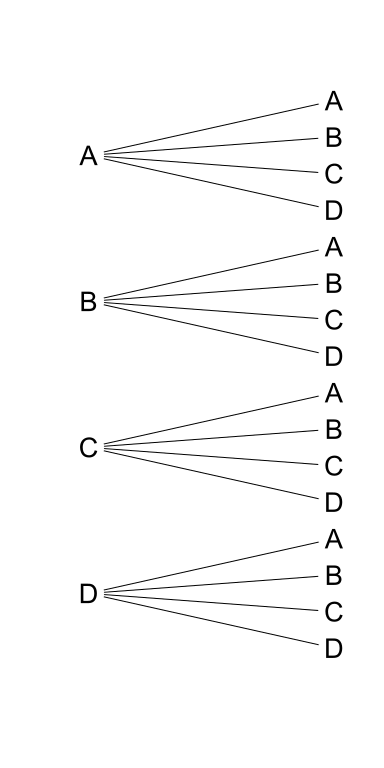## Sequences with replacement

### Each path represents a sequence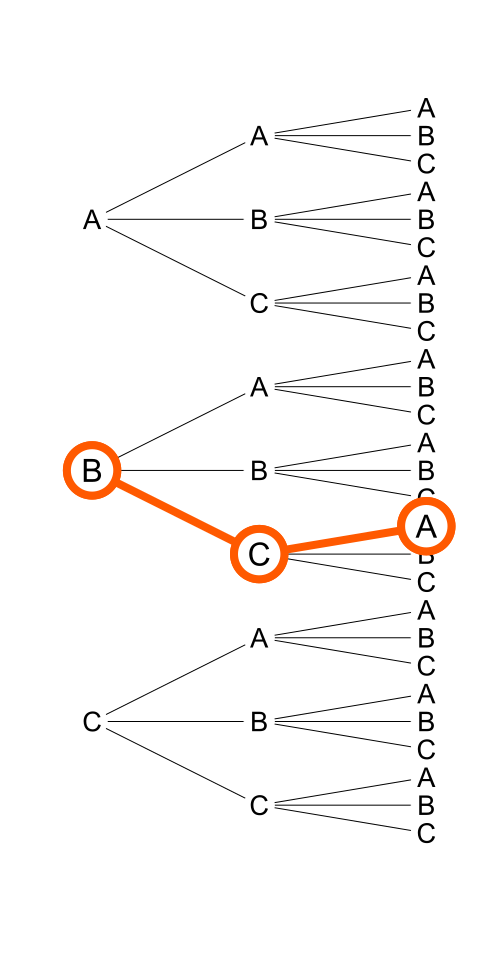## Sequences with replacement

### The total number of paths is the product of the number of candidate cards at each draw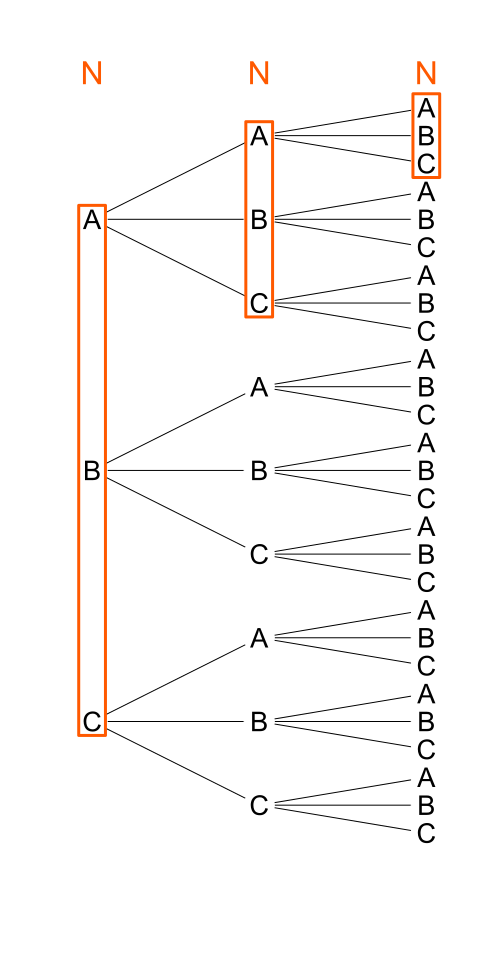## Sequences with replacement

### The total number of paths is the product of the number of candidate cards at each draw

$\underbrace{N \times N \times \cdots \times N}_{K \text{ draws}} = N^K$

## Sequences with replacement

### The total number of paths is the product of the number of candidate cards at each draw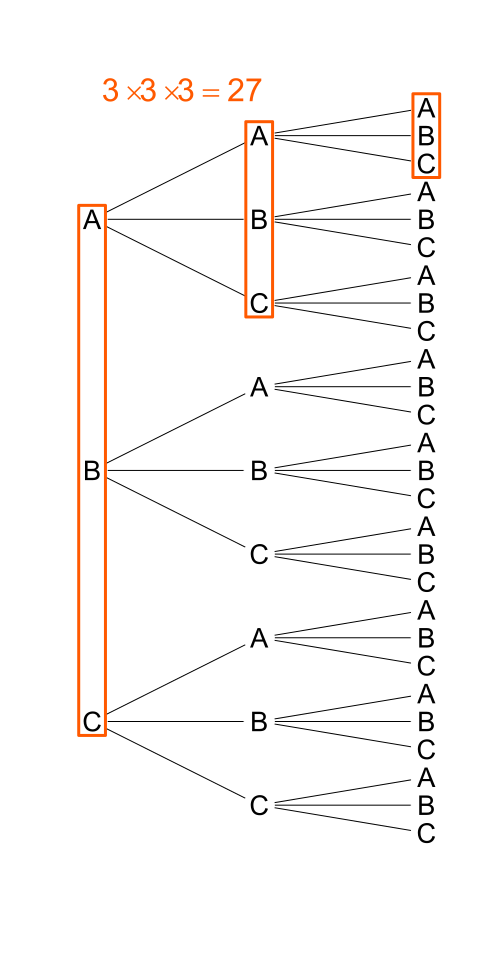## Sequences without replacement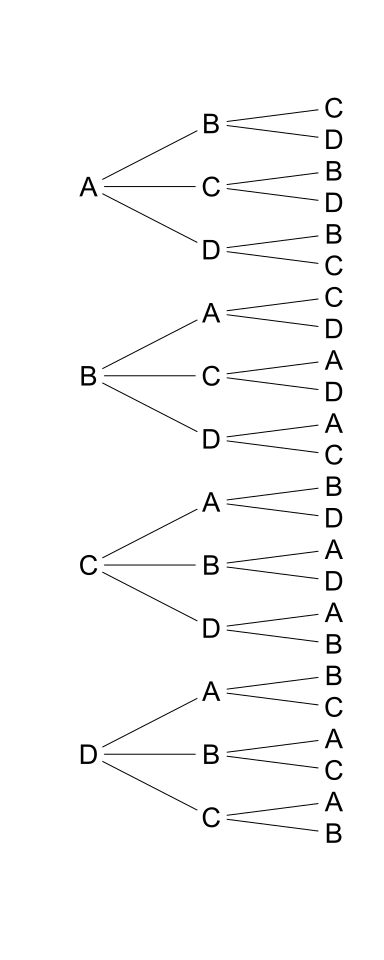## Sequences without replacement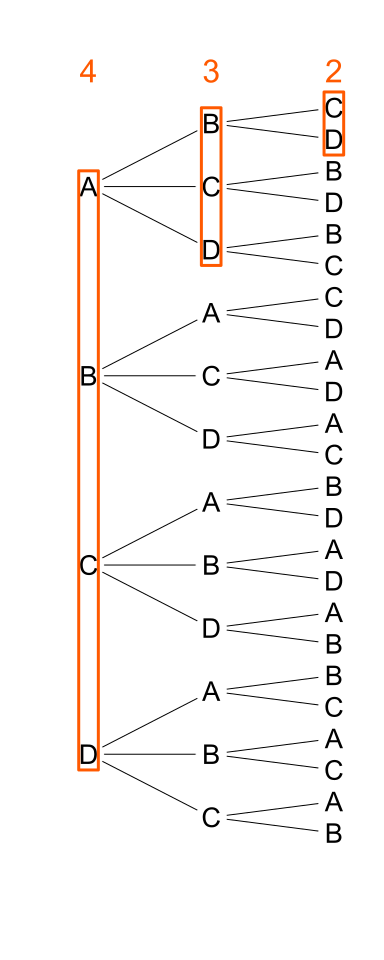## Sequences without replacement

### The total number of sequences is the product of the number of candidate cards at each draw

$\underbrace{N \times (N-1) \times \cdots \times (N-K+1)}_{K \text{ draws}} = \frac{N!}{(N-K)!}$

## The factorial

$N! = N\times(N-1)\times(N-2)\times \cdots \times 3 \times 2 \times 1$

$5! = 5 \times 4 \times 3 \times 2 \times 1$

## The factorial

In R:

factorial(5)
##  120

## The factorial

Note

$0! = 1$

## For sequences of K draws from N cards

• Total number of sequences is $$N^K$$ when drawing with replacement
• Total number of sequences is $$N!/(N-K)!$$ when drawing without replacement

## Hands

• In a sequence of draws, order matters:

$ABC \neq CAB$

## Hands

• In a hand (or sample) of draws, order does NOT matter:

$ABC = CAB$

## Hand without replacement

Go back to all the sequences:## Hand without replacement

### Which are duplicates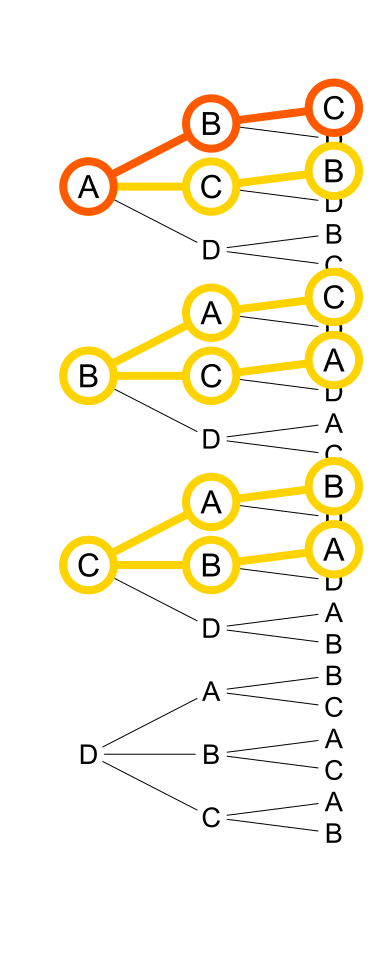## Hand without replacement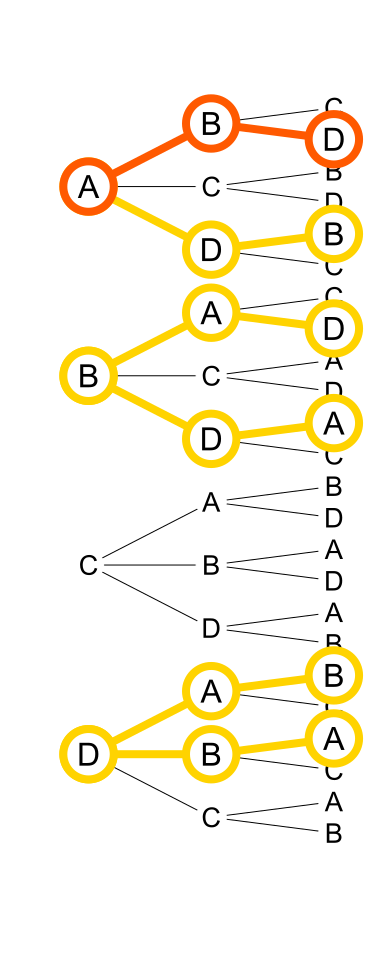## Hand without replacement

• For each hand there are a certain number of duplicate sequences

## Hand without replacement

• For each hand, how many sequences are there?

## Hand without replacement

• For each hand, how many sequences are there?
• Hint: Think of the hand as a mini deck.

## Hand without replacement

• For each hand, how many sequences are there?
• Hint: Think of the hand as a mini deck.
• How many sequences of $$K$$ cards from a deck of $$K$$ cards are there, without replacement?

## Hand without replacement

• For each hand, how many sequences are there?
• Hint: Think of the hand as a mini deck.
• How many sequences of $$K$$ cards from a deck of $$K$$ cards are there, without replacement?
• $$K\times(K-1)\times \cdots \times 2 \times 1$$

## Hand without replacement## Hand without replacement

• $$K$$ cards from $$N$$ without replacement has $$N!/(N-K)!$$ possible sequences
• For each hand there are $$K!$$ sequences (The hand to sequence multiplier)

## Hand without replacement

• To get the number of hands, divide the number of sequences by the multiplier.

$\frac{\text{Number sequences}}{\text{hand to sequence multiplier}} = \frac{\frac{N!}{(N-K)!}}{K!} = \frac{N!}{(N-K)!K!}$

## choose

${N \choose K} = \frac{N!}{(N-K)!K!}$

The number of hands of size $$K$$ from a deck of $$N$$ cards when drawing without replacement.

## choose

In R

#The number of 5 card hands from a 52 card deck
choose(52,5)
##  2598960

## Bernoulli random variable

• A process or experiment that generates a binary outcome (0 or 1; heads or tails; success or failure)

• Successive replications of the process are independent

• The probability $$P(outcome = 1)$$ is constant

• Notation:

• $$p = P(outcome = 1)$$
• $$q = (1-p) = P(outcome = 0)$$

## Bernoulli sequences

$Success,\ Success,\ Failure,\ Success$ $1,\ 0,\ 1,\ 1,\ 1,\ 0$ $tails,\ tails,\ tails,\ heads$

## Bernoulli sequences

Note: A Bernoulli sequence can be thought of as $$K$$ draws with replacement from a deck of $$N=2$$ cards.

## Bernoulli sequences

Because successive outcomes are independent and $$p$$ is constant,

\begin{align*}P(1,\ 0,\ 1,\ 1,\ 1,\ 0) &= P(1)P(0)P(1)P(1)P(1)P(0) \\ & = p(1-p)ppp(1-p) \\ & = p^4(1-p)^2 \end{align*}

## Binomial random variable

• The number of successes in a Bernoulli sequence

• Bernoulli properties still apply: independent outcomes, constant probability

• Notation:

• $$p$$ = probability of success in a single Bernoulli replicate
• $$N$$ = number of replicates in Bernoulli sequence

## Binomial random variable

• For a sequence of 10 coin flips, what are the possible number of heads?

## Binomial random variable

• For a sequence of 10 coin flips, what are the possible number of heads?
• 0, 1, 2, 3, …, 10

## Binomial random variable

• How do we calculate P(2 heads in 4 flips)?

## Binomial random variable

• How do we calculate P(2 heads in 4 flips)?
• If the sequence was $$H,\ H,\ T,\ T$$, then $P(H,\ H,\ T,\ T) = p^2(1-p)^2$

## Binomial random variable

• How do we calculate P(2 heads in 4 flips)?
• If the sequence was $$H,\ H,\ T,\ T$$, then $P(H,\ H,\ T,\ T) = p^2(1-p)^2$
• If the sequence was $$T,\ T,\ H,\ H$$, then $P(T,\ T,\ H,\ H) = p^2(1-p)^2$

## Binomial random variable

• How do we calculate P(2 heads in 4 flips)?
• We could list all possible 4 flip sequences …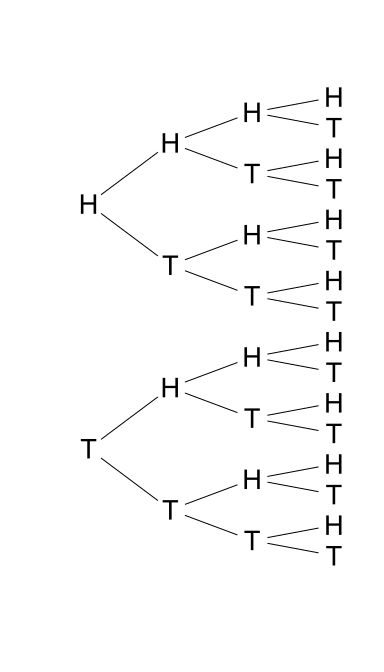## Binomial random variable

• How do we calculate P(2 heads in 4 flips)?
• We could list all possible 4 flip sequences …
• Identify all the sequences that have 2 heads …